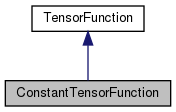Chombo + EB + MF  3.2
ConstantTensorFunction Class Reference

`#include <ConstantTensorFunction.H>`

Inheritance diagram for ConstantTensorFunction:[legend]

## Public Member Functions

ConstantTensorFunction (const RealTensor &a_value)

~ConstantTensorFunction ()
Destructor. More...

RealTensor operator() (const RealVect &a_x, Real a_t) constPublic Member Functions inherited from TensorFunction
TensorFunction (bool a_homogeneous, bool a_constant)

virtual ~TensorFunction ()
Destructor. More...

RealTensor operator() (const RealVect &a_x) const

bool isHomogeneous () const
Returns true if this function is homogeneous, false otherwise. More...

bool isConstant () const
Returns true if this function is constant, false otherwise. More...

## Private Attributes

RealTensor m_valueProtected Attributes inherited from TensorFunction
bool m_isHomogeneous

bool m_isConstant

## ◆ ConstantTensorFunction()

 ConstantTensorFunction::ConstantTensorFunction ( const RealTensor & a_value )
explicit

Creates a constant function returning the given value.

Parameters
 a_value The value returned by the function.

## ◆ ~ConstantTensorFunction()

 ConstantTensorFunction::~ConstantTensorFunction ( )

Destructor.

## ◆ operator()()

 RealTensor ConstantTensorFunction::operator() ( const RealVect & a_x, Real a_t ) const
virtual

Override this method to evaluate this function at the given point in space and time.

Parameters
 a_x A point in-dimensional space. a_t The time at which the function is evaluated.

Implements TensorFunction.

## ◆ m_value

 RealTensor ConstantTensorFunction::m_value
private

The documentation for this class was generated from the following file: